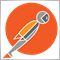# need help finding the highest OrderOpenPrice() to exclude it from OrderModify() - page 237

Marco vd Heijden:
It's working fine finding the highest order open price over here.

your part is working buddy but the OrderModify is the one not working , you got what i mean?369

What means Highest for you? Highest profit, highest entry, highest order ticket?

You said the OrderModify is not working. What is not working, describe what you experience, take screenshots, post logs.37

lippmaje:

What means Highest for you? Highest profit, highest entry, highest order ticket?

You said the OrderModify is not working. What is not working, describe what you experience, take screenshots, post logs.

ok buddy im using this code now ,

```double Modify()
{
double openprice=0;
int ticket;
double sl=0,tp=0;
for(int cnt=0;cnt<OrdersTotal();cnt++)
{

if(OrderSymbol()==Symbol()&&OrderMagicNumber()==Magic)
if(OrderType()==OP_SELL)
while(OrderOpenPrice()>openprice)
{
openprice=OrderOpenPrice();
ticket=OrderTicket();
}

if(OrderTicket()==ticket&&OrderType()==OP_SELL) {tp=0;}

else if(OrderType()==OP_SELL){ tp=OrderOpenPrice()-TakeProfit*Point;}
{

if(OrderType()==OP_SELL)
{
OrderModify(OrderTicket(),OrderOpenPrice(),sl,tp,0,Red);
}

}
} return (ticket); return(openprice);
}
```

what i need is to give all sell orders a take profit target except the highest one, highest in position, the open price of it is highest then the others.

this code give a take profit target for the new order opened if its not highest one , but if a new highest sell opened it wont modify the previous high, im getting OrderModify error 1. i guess its not updating the new highest sell order ... so far2380

shartouny:

ok buddy im using this code now ,

what i need is to give all sell orders a take profit target except the highest one, highest in position, the open price of it is highest then the others.

this code give a take profit target for the new order opened if its not highest one , but if a new highest sell opened it wont modify the previous high, im getting OrderModify error 1. i guess its not updating the new highest sell order ... so far

I just ran your code through an auto-formatter. Tell me if this makes sense.

```double Modify()
{
double openprice = 0;
int ticket;
double sl = 0, tp = 0;
for (int cnt = 0; cnt < OrdersTotal(); cnt++)
{
if (OrderSelect(cnt, SELECT_BY_POS, MODE_TRADES))
if (OrderSymbol() == Symbol() && OrderMagicNumber() == Magic)
if (OrderType() == OP_SELL)
while (OrderOpenPrice() > openprice)
{
openprice = OrderOpenPrice();
ticket = OrderTicket();
}

if (OrderTicket() == ticket && OrderType() == OP_SELL)
{
tp = 0;
}

else if (OrderType() == OP_SELL)
{
tp = OrderOpenPrice() - TakeProfit * Point;
}
{

if (OrderType() == OP_SELL)
{
OrderModify(OrderTicket(), OrderOpenPrice(), sl, tp, 0, Red);
}
}
}
return (ticket);
return (openprice);
}```37

nicholi shen:

I just ran your code through an auto-formatter. Tell me if this makes sense.

no difference buddy thanks anw